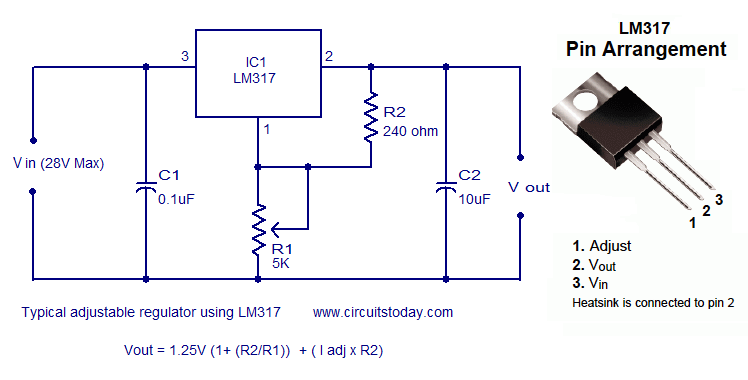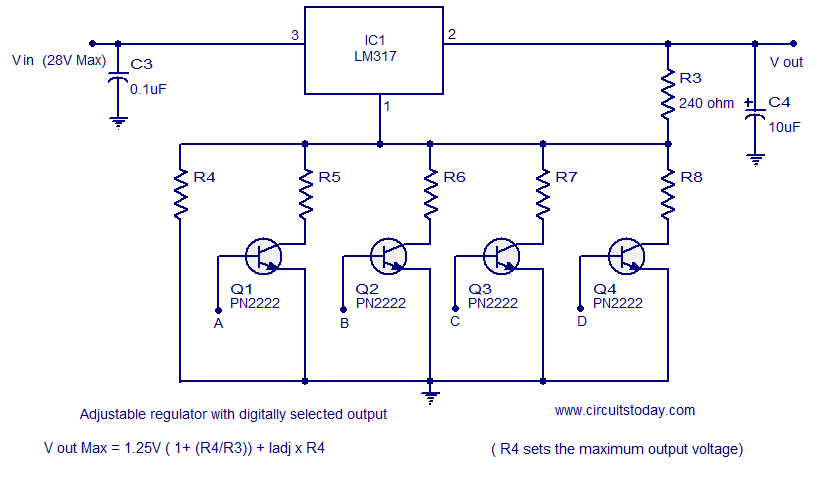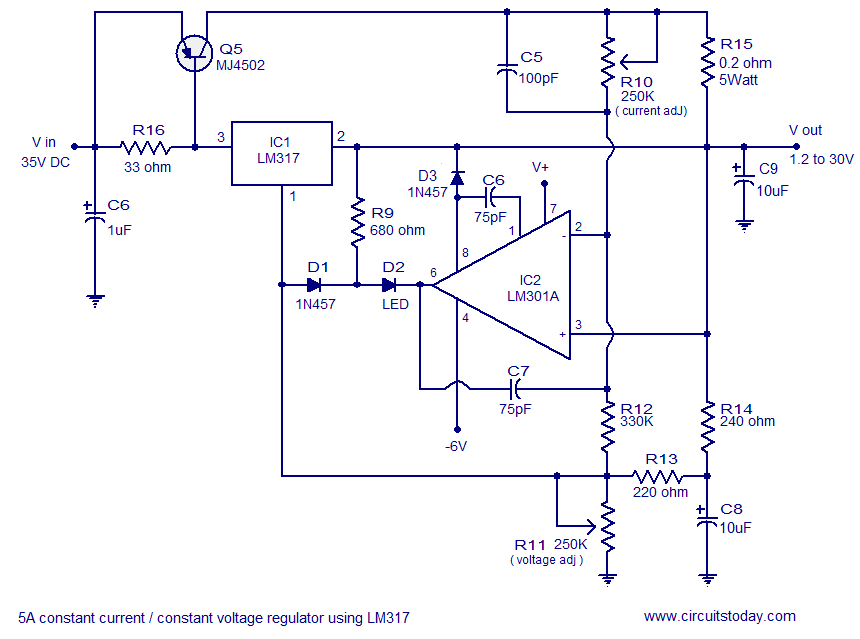## Audio

[Audio][slideshow]

## Some circuits based on LM317 voltage regulator

Few useful circuits using the voltage regulator IC LM317 is shown here. LM317 is a three terminal voltage regulator IC from National Semiconductors. The IC is capable of delivering up to 1A of output current. Input voltage can be up to 40V and output voltage can be adjusted from 1.2V to 37V.

### Typical positive voltage regulator circuit using LM317.A classic voltage regulator circuit using LM317 is shown above. Input voltage is fed to the pin3 (v in) of the IC and regulated output voltage is available from pin 2 ( V out) of the IC. Resistor network comprising of R1 and R2 connected in association to the pin1 (adj) is used to set the output voltage. C1 is the input filter capacitor while C2 is the output filter capacitor. The output voltage of the regulator circuit depends on the equation, Vout = 1.25V (1 + (R2/R1)) + I adj R2.

### Adjustable regulator with digitally selected output.LM317 voltage regulator with digitally selected output

A very simple adjustable regulator circuit with digitally selected output is shown above. The circuit is a just a modification of the ordinary voltage regulator using LM317. Parallel to the resistor R4, four more resistor branches each with a transistor switch is added and these resistors can be included or excluded in to the circuit by making the corresponding switching transistor ON. In simple words the output voltage will be according to the logic level of the digital inputs A, B, C and D. Logic high at terminal A will make Q1 ON and so resistor R5 will be added parallel to R4 and so on. Addition of each resistance in parallel to the R4 will reduce the effective resistance of the path and so the output voltage of the resistance will decrease in steps. The width of each step depends on the value of the resistors you choose. Resistor R4 sets the maximum output voltage and it is according to the equation V out Max = 1.25V (1 + (R4/R3)) + (Iadj x R4).

### 5A constant current constant voltage regulator.5A constant current constant voltage regulator

The circuit shown above is of a 5A constant current / constant voltage regulator using LM317. Such a circuit is a very inevitable gadget on the work bench of an electronic enthusiast. In addition to LM317 , LM310 a single operational amplifier is also used in the circuit. Diode D3 and capacitor C3 forms a compensation circuitry for the opamp. The output voltage of the regulator circuit is fed back to the non inverting input of the opamp while output voltage of the opamp is fed back to the inverting input of the opamp itself through capacitor C7. Resistor R16 limits the input current to the LM317 and base current to the transistor Q5. C6 is the input filter capacitor while C9 is the output filter capacitor. POT R10 can be used to adjust the output current while POT R11 can be used to adjust the output voltage. LED D2 provides a visual indication when the circuit is running in the constant current mode.

### Power follower circuit using LM317.

A voltage follower circuit is a circuit which produces considerable current gain while the voltage gain is maintained unity (or close to it). A power follower is nothing but a voltage follower with high current capability. A typical voltage follower circuit designed using a small signal transistor can handle few hundreds milli amperes of current. The power follower circuit shown below can handle up to 600mA of output current. The circuit shown below is nothing but a emitter follower circuit using power transistor LM195 (Q6) with a current limiter circuit based on LM317 is connected at the emitter. In simple words the current limiting circuit replaces the “emitter resistance” of a classic transistor emitter follower. Capacitor C10 is the input filter. LM195 is a monolithic power transistor with complete overload protection.Power follower circuit

Notes.

• All circuits shown above can be wired on perf board.
• Any way a PCB is the better option if you can make it.
• Maximum load current LM317 can handle is 1A.
• A heat sink must be fitted to the LM317 in all applications where the output current exceeds 250mA.
• The heat sink can be a 2 x 2 x 2 cm Finned Aluminium type.
• LM195 also requires a similar heat sink.
• Use a holder for mounting LM301.
• MJ4502 requires a 6 x 6 x 2 cm Finned Aluminium type heat sink.
• The dimensions of the heat sink are just approximate values and you can use slightly bigger or smaller ones according to the availability. Always bigger is better and there is nothing good a heat sink as large as possible.
• Input and output filter capacitors in these circuits are preferred to be Solid tantalum type.

[Lights][grids]